# Introduction

Simulating artifical tracks using mathematical or statistical models can help interpret the data from a tracking experiment. This tutorial will explain how to use simulation methods provided with the package. The simulation methods provided in the package are:

• brownianTrack() : a random walk in n dimensions.
• beaucheminTrack() : a special version of a random walk model specifically designed to describe T cell motion in an uninflamed lymph node.
• bootstrapTrack() : a data-driven simulation, where step speeds and turning angles are sampled from those observed in experimental data of interest.

# Datasets

First load the package:

library( celltrackR )
library( ggplot2 )


The package contains a dataset of T cells imaged in a mouse peripheral lymph node using two photon microscopy. We will here use this dataset as an example of how we can compare real tracking data with data from a simulation model.

The dataset consists of 21 tracks of individual cells in a tracks object:

str( TCells, list.len = 3 )

## List of 22
##  $0 : num [1:11, 1:4] 0 27.8 55.5 83.3 111.1 ... ## ..- attr(*, "dimnames")=List of 2 ## .. ..$ : chr [1:11] "1" "2" "3" "4" ...
##   .. ..$: chr [1:4] "t" "x" "y" "z" ##$ 1 : num [1:11, 1:4] 0 27.8 55.5 83.3 111.1 ...
##   ..- attr(*, "dimnames")=List of 2
##   .. ..$: chr [1:11] "1" "2" "3" "4" ... ## .. ..$ : chr [1:4] "t" "x" "y" "z"
##  $2 : num [1:39, 1:4] 0 27.8 55.5 83.3 111.1 ... ## ..- attr(*, "dimnames")=List of 2 ## .. ..$ : chr [1:39] "1" "2" "3" "4" ...
##   ..$: chr [1:4] "t" "x" "y" "z"  Note that this returns a single track matrix, which we can turn into a track object by using wrapTrack(): plot( wrapTrack(brownian) )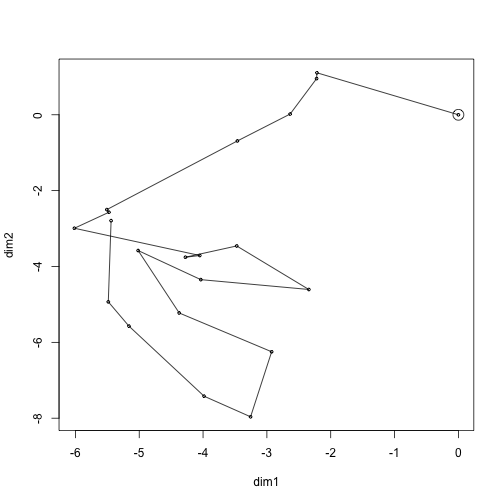We can also simulate multiple tracks at once using the simulateTracks() function. Do this and compare to the T cell tracks: brownian.tracks <- simulateTracks( 10, brownianTrack( nsteps = 20, dim = 3 ) ) par(mfrow=c(1,2)) plot( brownian.tracks, main = "simulated random walk" ) plot( normalizeTracks( TCells ), main = "real T cell data" )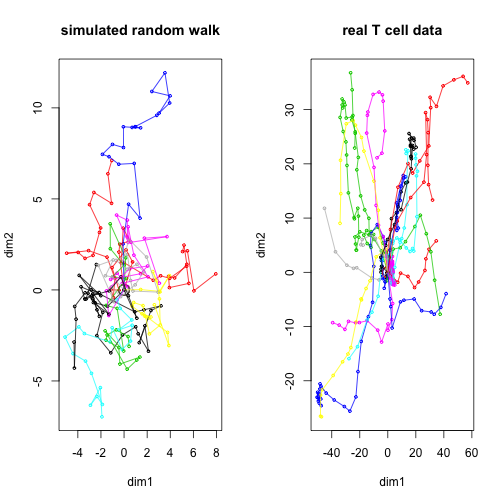### 1.2 Matching displacement to data In this simulation, the displacement vector for each step is sampled randomly in each dimension from a normal distribution of $$\mu=0, \sigma=1$$. To match the average displacement observed in the T cell data: # get displacement vectors step.displacements <- t( sapply( subtracks(TCells,1), displacementVector ) ) # get mean and sd of displacement in each dimension step.means <- apply( step.displacements, 2, mean ) step.sd <- apply( step.displacements, 2, sd ) # simulate brownian motion with the same statistics brownian.tracks.matched <- simulateTracks( 10, brownianTrack( nsteps = 20, dim = 3, mean = step.means, sd = step.sd ) ) # compare displacement distributions data.displacement <- sapply( subtracks( TCells,1), displacement ) matched.displacement <- sapply( subtracks( brownian.tracks.matched, 1 ), displacement ) df <- data.frame( disp = data.displacement, data = "TCells" ) df2 <- data.frame( disp = matched.displacement, data = "model" ) df <- rbind( df, df2 ) ggplot( df, aes( x = data, y = disp ) ) + geom_boxplot() + theme_classic()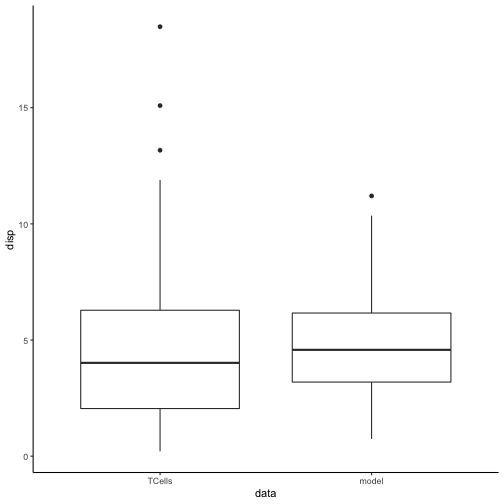# Plot new simulation versus real data par(mfrow=c(1,2)) plot( brownian.tracks.matched, main = "simulated random walk" ) plot( normalizeTracks( TCells ), main = "real T cell data" )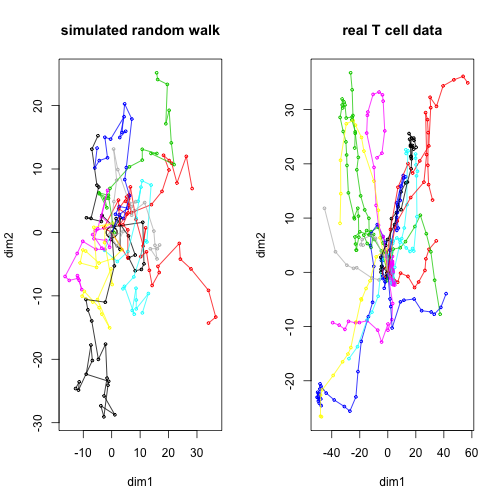### 1.3 A biased random walk We can bias movement in a certain direction by setting the mean in each dimension: # simulate brownian motion with bias brownian.tracks.bias <- simulateTracks( 10, brownianTrack( nsteps = 20, dim = 3, mean = c(1,1,1) ) ) plot( brownian.tracks.bias, main = "biased random walk" )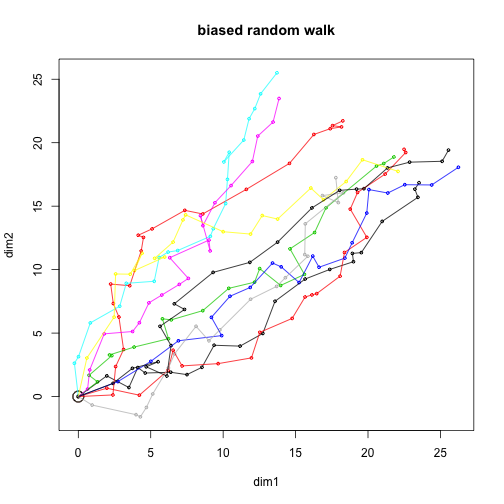# 2 The Beauchemin model of lymphocyte migration Aside from a simple random walk, the package also implements a slightly different model proposed by Beauchemin et al (2007). In contrast to the simple random walk, this model has a tuneable amount of persistence, and the cell pauses briefly between each step as it needs to reorient itself, which takes some time. For these reasons, the Beauchemin model generates tracks that are a bit more realistic than a simple random walk. beauchemin.tracks <- simulateTracks( 10, beaucheminTrack(sim.time=20) ) plot( beauchemin.tracks )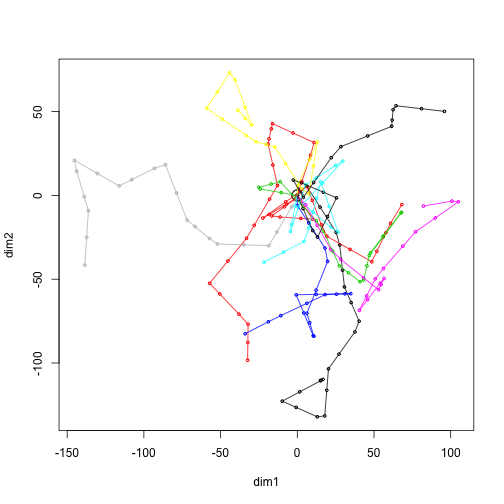See ?beaucheminTrack for further details. # 3 Bootstrapping method for simulating migration The final simulation method provided in the package is bootstrapTrack(), which does not assume an underlying migration model but samples speeds and turning angles from a real dataset. For example, we can do this for the T cell data: bootstrap.tracks <- simulateTracks( 10, bootstrapTrack( nsteps = 20, TCells ) ) plot( bootstrap.tracks )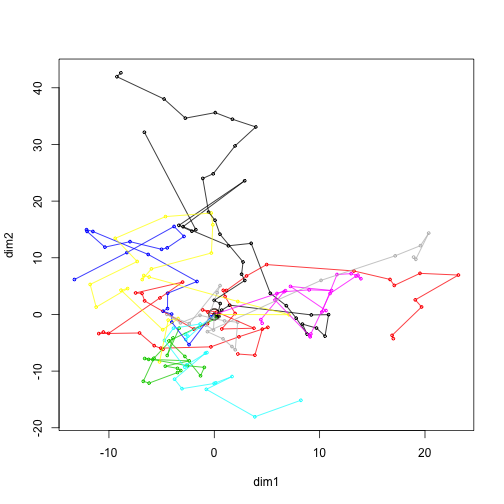If all is as should be, the distribution of speed and turning angles should now match the real data closely. Check this: # Simulate more tracks to reduce noice bootstrap.tracks <- simulateTracks( 100, bootstrapTrack( nsteps = 20, TCells ) ) # Compare step speeds in real data to those in bootstrap data real.speeds <- sapply( subtracks( TCells,1 ), speed ) bootstrap.speeds <- sapply( subtracks( bootstrap.tracks,1), speed ) dspeed <- data.frame( tracks = c( rep( "data", length( real.speeds ) ), rep( "bootstrap", length( bootstrap.speeds ) ) ), speed = c( real.speeds, bootstrap.speeds ) ) # Same for turning angles real.angles <- sapply( subtracks( TCells,2 ), overallAngle, degrees = TRUE ) bootstrap.angles <- sapply( subtracks( bootstrap.tracks,2), overallAngle, degrees = TRUE ) dangle <- data.frame( tracks = c( rep( "data", length( real.angles ) ), rep( "bootstrap", length( bootstrap.angles ) ) ), angle = c( real.angles, bootstrap.angles ) ) # plot pspeed <- ggplot( dspeed, aes( x = tracks, y = speed ) ) + geom_violin( color = NA, fill = "gray" ) + geom_boxplot( width = 0.3 ) + theme_classic() pangle <- ggplot( dangle, aes( x = tracks, y = angle ) ) + geom_violin( color = NA, fill = "gray" ) + geom_boxplot( width = 0.3 ) + theme_classic() gridExtra::grid.arrange( pspeed, pangle, ncol = 2 )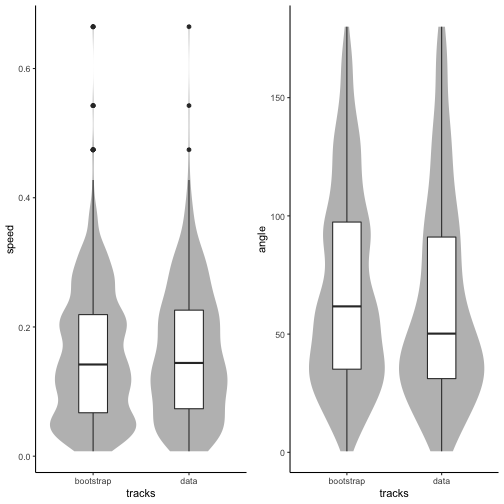# 4 Example: Comparing data with models ### 4.1 Mean square displacement plot To compare two different models with the real T cell data, we make a mean square displacement plot. To remove the effect of noise, we simulate a greater number of tracks than before: # Simulate more tracks brownian.tracks <- simulateTracks( 100, brownianTrack( nsteps = 20, dim = 3, mean = step.means, sd = step.sd ) ) bootstrap.tracks <- simulateTracks( 100, bootstrapTrack( nsteps = 20, TCells ) ) msd.data <- aggregate( TCells, squareDisplacement, FUN = "mean.se" ) msd.data$data <- "data"
msd.brownian <- aggregate( brownian.tracks, squareDisplacement, FUN = "mean.se" )
msd.brownian$data <- "brownian" msd.bootstrap <- aggregate( bootstrap.tracks, squareDisplacement, FUN = "mean.se" ) msd.bootstrap$data <-"bootstrap"

msd <- rbind( msd.data, msd.brownian, msd.bootstrap )
ggplot( msd, aes( x = i, y = mean, ymin = lower, ymax = upper, color = data, fill = data ) ) +
geom_ribbon( color= NA, alpha  = 0.2 ) +
geom_line() +
labs( x = "t (steps)",
y = "square displacement" ) +
scale_x_continuous(limits= c(0,20) ) +
theme_bw()

## Warning: Removed 18 rows containing missing values (geom_path).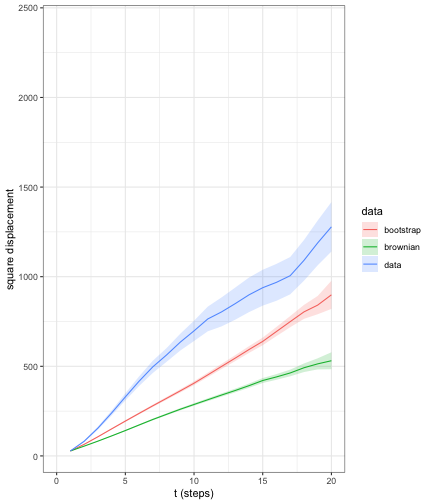Both the random walk model and the bootstrapped tracks underestimate the MSD as observed in real data - even though the bootstrapped data at least matches speed and turning angle distributions well.

This suggests there is more directional persistence in the real data than those models assume. In the next section, we will check this by making the autocorrelation plot.

### 4.2 Persistence: autocovariance plot

To check for directional persistence, we generate an autocovariance plot:

# compute autocorrelation
acor.data <- aggregate( TCells, overallDot, FUN = "mean.se" )
acor.data$data <- "data" acor.brownian <- aggregate( brownian.tracks, overallDot, FUN = "mean.se" ) acor.brownian$data <- "brownian"
acor.bootstrap <- aggregate( bootstrap.tracks, overallDot, FUN = "mean.se" )
acor.bootstrap\$data <-"bootstrap"

acor <- rbind( acor.data, acor.brownian, acor.bootstrap )
ggplot( acor, aes( x = i, y = mean, ymin = lower, ymax = upper, color = data, fill = data ) ) +
geom_ribbon( color= NA, alpha  = 0.2 ) +
geom_line() +
labs( x = "dt (steps)",
y = "autocovariance" ) +
scale_x_continuous(limits= c(0,20) ) +
theme_bw()

## Warning: Removed 18 rows containing missing values (geom_path).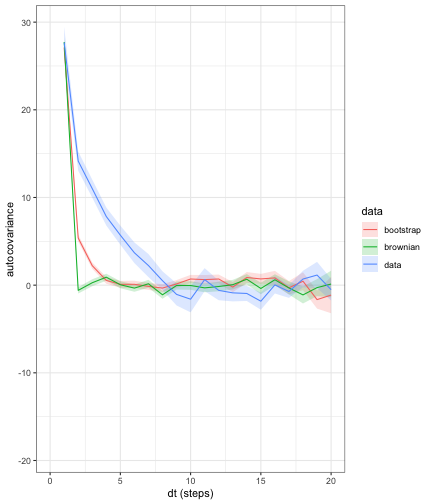Indeed, the autocovariance drops less steeply for the real T cell data, which indicates that there is a directional persistence in the T cell data that is not captured by the brownian and bootstrapping models. Thus, even when a model captures some aspects of the walk statistics in the data, it may still behave differently in other respects.